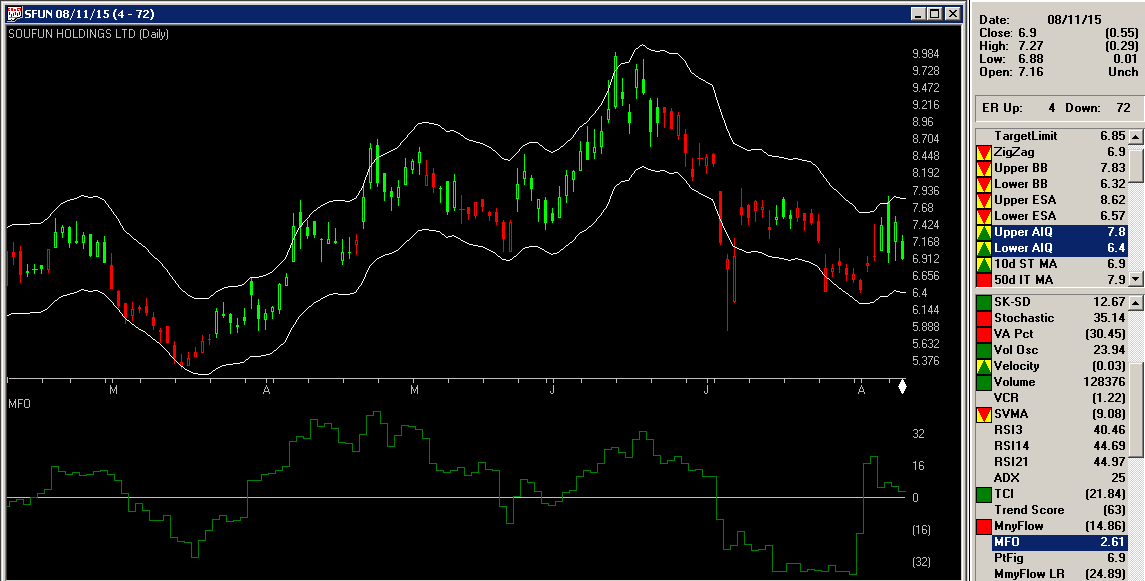# Vitali Apirine’s – The Money Flow Oscillator

The AIQ code I am providing here is based on Vitali Apirine’s article in October issue of Stocks & Commodities, “The Money Flow Oscillator.” This code displays an indicator based on Apirine’s money flow oscillator (MFO), an example of which is shown in Figure 7.FIGURE 7: AIQ. Here is an example of the MFO(20) indicator on a chart of SFUN.
```!THE MONEY FLOW OSCILLATOR
!Author: Vitali Aprine, TASC October 2015
!Coded by: Richard Denning, 8/11/15

!INPUTS:
MFOlen is 20.
H is [high].
H1 is valresult(H,1).
L is [low].
L1 is valresult([low],1).
V is [volume].

!INDICATOR CODE:
MFmult is ((H-L1)-(H1-L))/((H-L1)+(H1-L)).
MFvol is MFmult*V.
MFO is sum(MFvol,MFOlen)/sum(V,MFOlen)*100. !PLOT
```
—Richard Denning